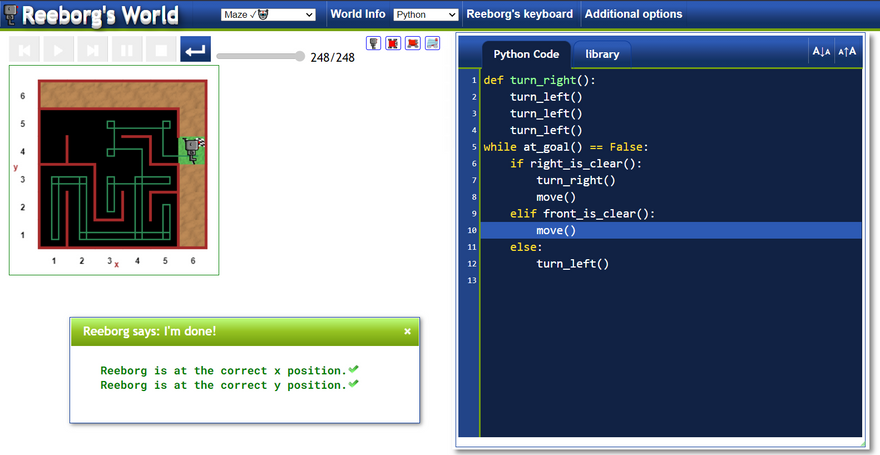## CodeNewbie Community 🌱

Posted on • Originally published at bharadwaj.hashnode.dev on

# Reeborgs robot in Python

Yesterday, I have seen one beautiful website called Roobergs world. Where there will be a robot called Rooberg which is almost similar to the widely known Karl robot. The advantage of this is we can write the code in python in order to solve those riddles.

In this blog, I am going to discuss Five riddles which not only give a fun but also cover some important topics in Python say functions. code blocks and while loops.

To get then riddles, Go to the browser and search Reeborgs world hurdles and you can practice the code there.

Let's start with Hurdle 1 (you can select it in the first dropdown menu) and make sure to select python in the second dropdown. You can also check world info which gives the information about what are the functions to be used and how to write the code and also shows the output format. You can also see the Reeborgs keyboard beside python which gives information about the pre-built functions of Reeborg.

Hurdle 1

The image of hurdle 1 is as follows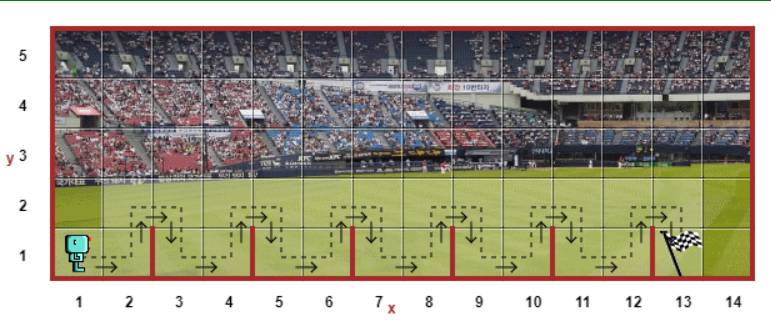Our robot should stop its execution at the flag with correct coordinates. If you examine the Reeborgs keyboard correctly you can see that there is no turn_right function. To successfully complete this riddle we need turn_right so let's first define it.

``````def turn_right():
turn_left()
turn_left()
turn_left()

``````

Next whenever there is a wall then we should be able to jump the wall. And we can clearly see that distance between the walls is pretty the same which makes our work far easier. The code for it is

``````def jump():
move()
turn_left()
move()
turn_right()
move()
turn_right()
move()
turn_left()

``````

By executing the above code we can see that it only runs as many times as we call it and we should stop our execution once we reach the flag. To do that we use a while loop. Normally while loop requires some breakpoint. So, our breakpoint is the flag.

``````i = 0
while i<6:
jump()
i +=1

``````

Once you compile it according to the above code then you will get the output exactly and Congrats you just passed the first test.

The output screen is as follows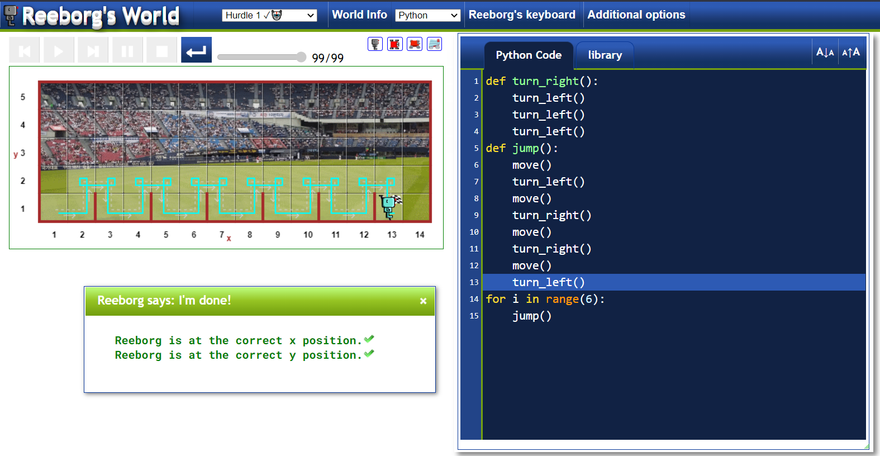Hurdle 2

The image of Hurdle 2 is as follows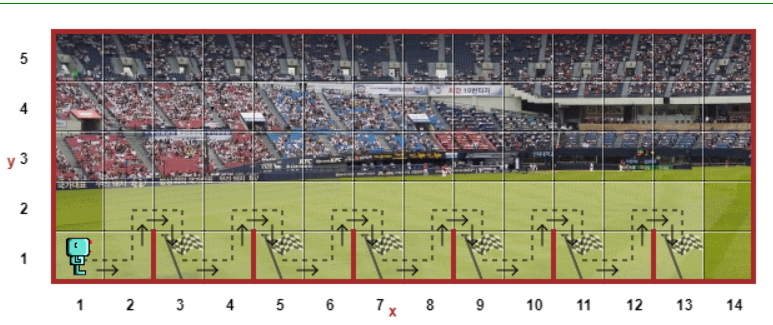The main difference between the previous one and this is the position of the flag is not constant and it can be anywhere. To tackle that we should use at_goal() function which is a pre-built function. The use of this is it indicates the position of the flag.

The code for this is as follows

``````def turn_right():
turn_left()
turn_left()
turn_left()
def jump():
move()
turn_left()
move()
turn_right()
move()
turn_right()
move()
turn_left()
while at_goal() == False:
jump()

``````

Since we don't know the exact position of the flag, in spite of 6 we use at_goal() function here. That's it we have completed Hurdle 2 also.

The output screen is as follows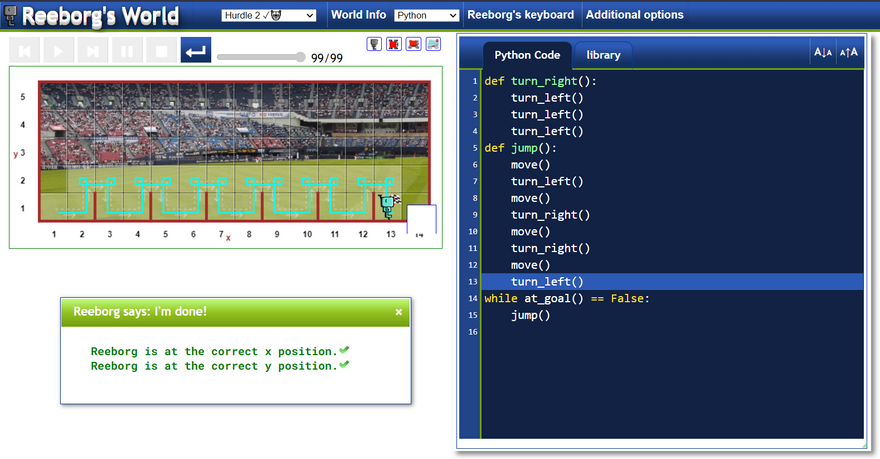Hurdle 3

The image of Hurdle 3 is as follows (it may not be the same for everyone)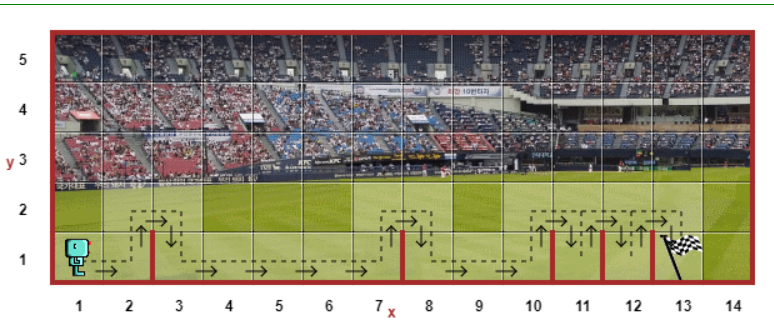Here we can see that the position of the walls is not constant but the position of the flag is constant. In the world info, we can see two new functions front_is_clear() and wall_in_front(). By their names, we can guess that they denote front is clear and wall in front. By using these functions we can write the code for Hurdle 3.

``````def turn_right():
turn_left()
turn_left()
turn_left()
def jump():
turn_left()
move()
turn_right()
move()
turn_right()
move()
turn_left()
while at_goal() == False:
if wall_in_front():
jump()
else:
move()

``````

We should jump only if there is a wall in front. And if there is no wall then we should simply move that's it.

The output screen is as follows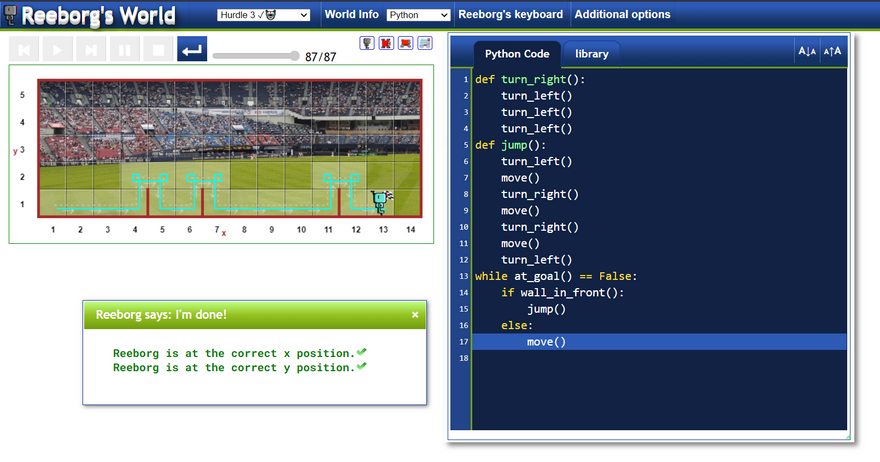Hurdle 4

The image of it is as follows: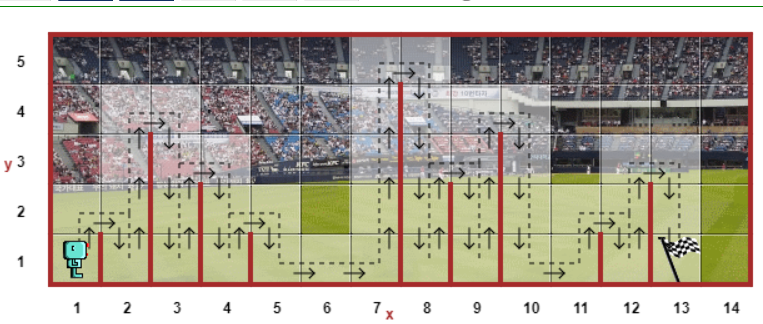Here you can see that the size of the walls is not fixed and also their places are also not fixed. In the Reeborgs keyboard, under conditions section, we can see wall_on_right() function. It just checks whether there is a wall on its right side or not. By using this function we can solve this riddle.

``````def turn_right():
turn_left()
turn_left()
turn_left()
def jump():
turn_left()
while wall_on_right():
move()
turn_right()
move()
turn_right()
while front_is_clear():
move()
turn_left()
while at_goal() == False:
if wall_in_front():
jump()
else:
move()

``````

The output screen is as follows

Maze Here the position of the walls and flag is fixed but not the position of Reeborg. The image for this is as follows

``````def turn_right():
turn_left()
turn_left()
turn_left()
while at_goal() == False:
if right_is_clear():
turn_right()
move()
elif front_is_clear():
move()
else:
turn_left()

``````

The output screen is as follows